Documentation

### This is machine translation

Mouseover text to see original. Click the button below to return to the English version of the page.

Note: This page has been translated by MathWorks. Click here to see
To view all translated materials including this page, select Country from the country navigator on the bottom of this page.

## Pricing American Basket Options by Monte Carlo Simulation

This example shows how to model the fat-tailed behavior of asset returns and assess the impact of alternative joint distributions on basket option prices. Using various implementations of a separable multivariate Geometric Brownian Motion (GBM) process, often referred to as a multi-dimensional market model, the example simulates risk-neutral sample paths of an equity index portfolio and prices basket put options using the technique of Longstaff & Schwartz.

In addition, this example also illustrates salient features of the Stochastic Differential Equation (SDE) architecture, including

• Customized random number generation functions that compare Brownian motion and Brownian copulas

• End-of-period processing functions that form an equity index basket and price American options on the underlying basket based on the least squares method of Longstaff & Schwartz

• Piecewise probability distributions and Extreme Value Theory (EVT)

This example also highlights important issues of volatility and interest rate scaling. It illustrates how equivalent results can be achieved by working with daily or annualized data. For more information about EVT and copulas, see Using Extreme Value Theory and Copulas to Evaluate Market Risk (Econometrics Toolbox).

### Overview of the Modeling Framework

The ultimate objective of this example is to compare basket option prices derived from different noise processes. The first noise process is a traditional Brownian motion model whose index portfolio price process is driven by correlated Gaussian random draws. As an alternative, the Brownian motion benchmark is compared to noise processes driven by Gaussian and Student's t copulas, referred to collectively as a Brownian copula.

A copula is a multivariate cumulative distribution function (CDF) with uniformly-distributed margins. Although the theoretical foundations were established decades ago, copulas have experienced a tremendous surge in popularity over the last few years, primarily as a technique for modeling non-Gaussian portfolio risks.

Although numerous families exist, all copulas represent a statistical device for modeling the dependence structure between two or more random variables. In addition, important statistics, such as rank correlation and tail dependence are properties of a given copula and are unchanged by monotonic transforms of their margins.

These copula draws produce dependent random variables, which are subsequently transformed to individual variables (margins). This transformation is achieved with a semi-parametric probability distribution with generalized Pareto tails.

The risk-neutral market model to simulate iswhere the risk-free rate, r, is assumed constant over the life of the option. Because this is a separable multivariate model, the risk-free return is a diagonal matrix in which the same riskless return is applied to all indices. Dividend yields are ignored to simplify the model and its associated data collection.

In contrast, the specification of the exposure matrix, sigma, depends on how the driving source of uncertainty is modeled. You can model it directly as a Brownian motion (correlated Gaussian random numbers implicitly mapped to Gaussian margins) or model it as a Brownian copula (correlated Gaussian or t random numbers explicitly mapped to semi-parametric margins).

Because the CDF and inverse CDF (quantile function) of univariate distributions are both monotonic transforms, a copula provides a convenient way to simulate dependent random variables whose margins are dissimilar and arbitrarily distributed. Moreover, because a copula defines a given dependence structure regardless of its margins, copula parameter calibration is typically easier than estimation of the joint distribution function.

Once you have simulated sample paths, options are priced by the least squares regression method of Longstaff & Schwartz (see Valuing American Options by Simulation: A Simple Least-Squares Approach, The Review of Financial Studies, Spring 2001). This approach uses least squares to estimate the expected payoff of an option if it is not immediately exercised. It does so by regressing the discounted option cash flows received in the future on the current price of the underlier associated with all in-the-money sample paths. The continuation function is estimated by a simple third-order polynomial, in which all cash flows and prices in the regression are normalized by the option strike price, improving numerical stability.

### Import the Supporting Historical Dataset

Load a daily historical dataset of 3-month Euribor, the trading dates spanning the interval 07-Feb-2001 to 24-Apr-2006, and the closing index levels of the following representative large-cap equity indices:

• TSX Composite (Canada)

• CAC 40 (France)

• DAX (Germany)

• Nikkei 225 (Japan)

• FTSE 100 (UK)

• S&P 500 (US)

clear load Data_GlobalIdx2 

The following plots illustrate this data. Specifically, the plots show the relative price movements of each index and the Euribor risk-free rate proxy. The initial level of each index has been normalized to unity to facilitate the comparison of relative performance over the historical record.

nIndices = size(Data,2)-1; % # of indices prices = Data(:,1:end-1); yields = Data(:,end); % daily effective yields yields = 360 * log(1 + yields); % continuously-compounded, annualized yield plot(dates, ret2tick(tick2ret(prices,[],'continuous'),[],[],[],'continuous')) datetick('x') xlabel('Date') ylabel('Index Value') title ('Normalized Daily Index Closings') legend(series{1:end-1}, 'Location', 'NorthWest') 
Warning: This syntax will be removed in a future release. See the documentation for recommended usage. Warning: This syntax will be removed in a future release. See the documentation for recommended usage.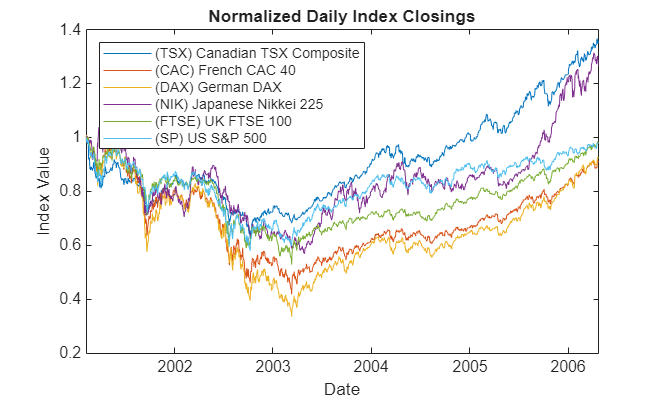plot(dates, 100 * yields) datetick('x') xlabel('Date') ylabel('Annualized Yield (%)') title('Risk Free Rate (3-Month Euribor Continuously-Compounded)')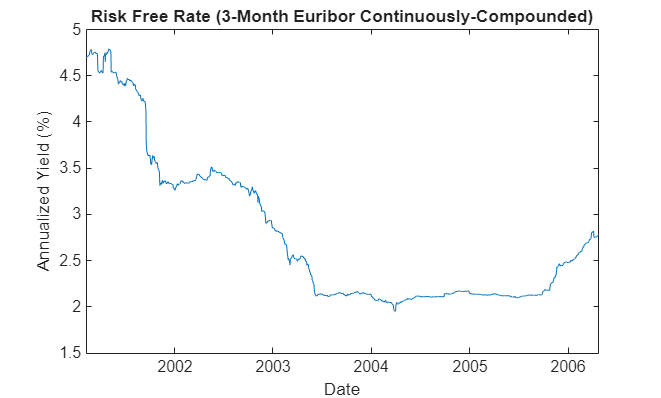### Extreme Value Theory & Piecewise Probability Distributions

To prepare for copula modeling, characterize individually the distribution of returns of each index. Although the distribution of each return series may be characterized parametrically, it is useful to fit a semi-parametric model using a piecewise distribution with generalized Pareto tails. This uses Extreme Value Theory to better characterize the behavior in each tail.

The Statistics and Machine Learning Toolbox™ software currently supports two univariate probability distributions related to EVT, a statistical tool for modeling the fat-tailed behavior of financial data such as asset returns and insurance losses:

• Generalized Extreme Value (GEV) distribution, which uses a modeling technique known as the block maxima or minima method. This approach, divides a historical dataset into a set of sub-intervals, or blocks, and the largest or smallest observation in each block is recorded and fitted to a GEV distribution.

• Generalized Pareto (GP) distribution, uses a modeling technique known as the distribution of exceedances or peaks over threshold method. This approach sorts a historical dataset and fits the amount by which those observations that exceed a specified threshold to a GP distribution.

The following analysis highlights the Pareto distribution, which is more widely used in risk management applications.

Suppose we want to create a complete statistical description of the probability distribution of daily asset returns of any one of the equity indices. Assume that this description is provided by a piecewise semi-parametric distribution, where the asymptotic behavior in each tail is characterized by a generalized Pareto distribution.

Ultimately, a copula will be used to generate random numbers to drive the simulations. The CDF and inverse CDF transforms will capture the volatility of simulated returns as part of the diffusion term of the SDE. The mean return of each index is governed by the riskless rate and incorporated in the drift term of the SDE. The following code segment centers the returns (that is, extracts the mean) of each index.

Because the following analysis uses extreme value theory to characterize the distribution of each equity index return series, it is helpful to examine details for a particular country:

returns = tick2ret(prices,[],'continuous'); % convert prices to returns returns = bsxfun(@minus, returns, mean(returns)); % center the returns index = 3; % Germany stored in column 3 plot(dates(2:end), returns(:,index)) datetick('x') xlabel('Date') ylabel('Return') title(['Daily Logarithmic Centered Returns: ' series{index}]) 
Warning: This syntax will be removed in a future release. See the documentation for recommended usage.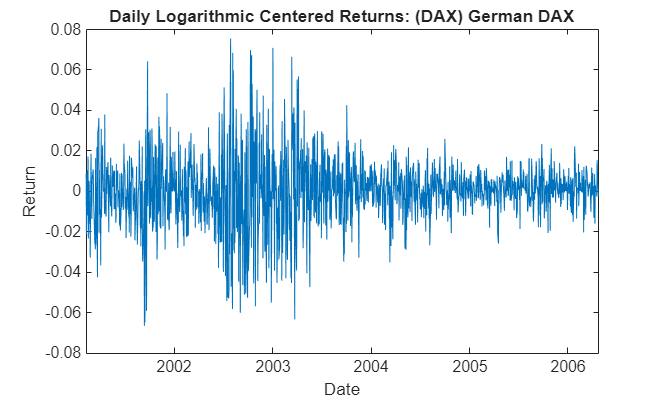Note that this code segment can be changed to examine details for any country.

Using these centered returns, estimate the empirical, or non-parametric, CDF of each index with a Gaussian kernel. This smoothes the CDF estimates, eliminating the staircase pattern of unsmoothed sample CDFs. Although non-parametric kernel CDF estimates are well-suited for the interior of the distribution, where most of the data is found, they tend to perform poorly when applied to the upper and lower tails. To better estimate the tails of the distribution, apply EVT to the returns that fall in each tail.

Specifically, find the upper and lower thresholds such that 10% of the returns are reserved for each tail. Then fit the amount by which the extreme returns in each tail fall beyond the associated threshold to a Pareto distribution by maximum likelihood.

The following code segment creates one object of type paretotails for each index return series. These Pareto tail objects encapsulate the estimates of the parametric Pareto lower tail, the non-parametric kernel-smoothed interior, and the parametric Pareto upper tail to construct a composite semi-parametric CDF for each index.

tailFraction = 0.1; % decimal fraction allocated to each tail tails = cell(nIndices,1); % cell array of Pareto tail objects for i = 1:nIndices tails{i} = paretotails(returns(:,i), tailFraction, 1 - tailFraction, 'kernel'); end 

The resulting piecewise distribution object allows interpolation within the interior of the CDF and extrapolation (function evaluation) in each tail. Extrapolation allows estimation of quantiles outside the historical record, which is invaluable for risk management applications.

Pareto tail objects also provide methods to evaluate the CDF and inverse CDF (quantile function), and to query the cumulative probabilities and quantiles of the boundaries between each segment of the piecewise distribution.

Now that three distinct regions of the piecewise distribution have been estimated, graphically concatenate and display the result.

The following code calls the CDF and inverse CDF methods of the Pareto tails object of interest with data other than that upon which the fit is based. The referenced methods have access to the fitted state. They are now invoked to select and analyze specific regions of the probability curve, acting as a powerful data filtering mechanism.

For reference, the plot also includes a zero-mean Gaussian CDF of the same standard deviation. To a degree, the variation in options prices reflect the extent to which the distribution of each asset differs from this normal curve.

minProbability = cdf(tails{index}, (min(returns(:,index)))); maxProbability = cdf(tails{index}, (max(returns(:,index)))); pLowerTail = linspace(minProbability , tailFraction , 200); % lower tail pUpperTail = linspace(1 - tailFraction, maxProbability , 200); % upper tail pInterior = linspace(tailFraction , 1 - tailFraction, 200); % interior plot(icdf(tails{index}, pLowerTail), pLowerTail, 'red' , 'LineWidth', 2) hold on grid on plot(icdf(tails{index}, pInterior) , pInterior , 'black', 'LineWidth', 2) plot(icdf(tails{index}, pUpperTail), pUpperTail, 'blue' , 'LineWidth', 2) limits = axis; x = linspace(limits(1), limits(2)); plot(x, normcdf(x, 0, std(returns(:,index))), 'green', 'LineWidth', 2) fig = gcf; fig.Color = [1 1 1]; hold off xlabel('Centered Return') ylabel('Probability') title (['Semi-Parametric/Piecewise CDF: ' series{index}]) legend({'Pareto Lower Tail' 'Kernel Smoothed Interior' ... 'Pareto Upper Tail' 'Gaussian with Same \sigma'}, 'Location', 'NorthWest')The lower and upper tail regions, displayed in red and blue, respectively, are suitable for extrapolation, while the kernel-smoothed interior, in black, is suitable for interpolation.

### Copula Calibration

The Statistics and Machine Learning Toolbox software includes functionality that calibrates and simulates Gaussian and t copulas.

Using the daily index returns, estimate the parameters of the Gaussian and t copulas using the function copulafit. Since a t copula becomes a Gaussian copula as the scalar degrees of freedom parameter (DoF) becomes infinitely large, the two copulas are really of the same family, and therefore share a linear correlation matrix as a fundamental parameter.

Although calibration of the linear correlation matrix of a Gaussian copula is straightforward, the calibration of a t copula is not. For this reason, the Statistics and Machine Learning Toolbox software offers two techniques to calibrate a t copula:

• The first technique performs maximum likelihood estimation (MLE) in a two-step process. The inner step maximizes the log-likelihood with respect to the linear correlation matrix, given a fixed value for the degrees of freedom. This conditional maximization is placed within a 1-D maximization with respect to the degrees of freedom, thus maximizing the log-likelihood over all parameters. The function being maximized in this outer step is known as the profile log-likelihood for the degrees of freedom.

• The second technique is derived by differentiating the log-likelihood function with respect to the linear correlation matrix, assuming the degrees of freedom is a fixed constant. The resulting expression is a non-linear equation that can be solved iteratively for the correlation matrix. This technique approximates the profile log-likelihood for the degrees of freedom parameter for large sample sizes. This technique is usually significantly faster than the true maximum likelihood technique outlined above; however, you should not use it with small or moderate sample sizes as the estimates and confidence limits may not be accurate.

When the uniform variates are transformed by the empirical CDF of each margin, the calibration method is often known as canonical maximum likelihood (CML). The following code segment first transforms the daily centered returns to uniform variates by the piecewise, semi-parametric CDFs derived above. It then fits the Gaussian and t copulas to the transformed data:

U = zeros(size(returns)); for i = 1:nIndices U(:,i) = cdf(tails{i}, returns(:,i)); % transform each margin to uniform end options = statset('Display', 'off', 'TolX', 1e-4); [rhoT, DoF] = copulafit('t', U, 'Method', 'ApproximateML', 'Options', options); rhoG = copulafit('Gaussian', U); 

The estimated correlation matrices are quite similar but not identical.

corrcoef(returns) % linear correlation matrix of daily returns 
ans = 1.0000 0.4813 0.5058 0.1854 0.4573 0.6526 0.4813 1.0000 0.8485 0.2261 0.8575 0.5102 0.5058 0.8485 1.0000 0.2001 0.7650 0.6136 0.1854 0.2261 0.2001 1.0000 0.2295 0.1439 0.4573 0.8575 0.7650 0.2295 1.0000 0.4617 0.6526 0.5102 0.6136 0.1439 0.4617 1.0000 
rhoG % linear correlation matrix of the optimized Gaussian copula 
rhoG = 1.0000 0.4745 0.5018 0.1857 0.4721 0.6622 0.4745 1.0000 0.8606 0.2393 0.8459 0.4912 0.5018 0.8606 1.0000 0.2126 0.7608 0.5811 0.1857 0.2393 0.2126 1.0000 0.2396 0.1494 0.4721 0.8459 0.7608 0.2396 1.0000 0.4518 0.6622 0.4912 0.5811 0.1494 0.4518 1.0000 
rhoT % linear correlation matrix of the optimized t copula 
rhoT = 1.0000 0.4671 0.4858 0.1907 0.4734 0.6521 0.4671 1.0000 0.8871 0.2567 0.8500 0.5122 0.4858 0.8871 1.0000 0.2326 0.7723 0.5877 0.1907 0.2567 0.2326 1.0000 0.2503 0.1539 0.4734 0.8500 0.7723 0.2503 1.0000 0.4769 0.6521 0.5122 0.5877 0.1539 0.4769 1.0000 

Note the relatively low degrees of freedom parameter obtained from the t copula calibration, indicating a significant departure from a Gaussian situation.

DoF % scalar degrees of freedom parameter of the optimized t copula 
DoF = 4.8613 

### Copula Simulation

Now that the copula parameters have been estimated, simulate jointly-dependent uniform variates using the function copularnd.

Then, by extrapolating the Pareto tails and interpolating the smoothed interior, transform the uniform variates derived from copularnd to daily centered returns via the inverse CDF of each index. These simulated centered returns are consistent with those obtained from the historical dataset. The returns are assumed to be independent in time, but at any point in time possess the dependence and rank correlation induced by the given copula.

The following code segment illustrates the dependence structure by simulating centered returns using the t copula. It then plots a 2-D scatter plot with marginal histograms for the French CAC 40 and German DAX using the Statistics and Machine Learning Toolbox scatterhist function. The French and German indices were chosen simply because they have the highest correlation of the available data.

nPoints = 10000; % # of simulated observations s = RandStream.getGlobalStream(); reset(s) R = zeros(nPoints, nIndices); % pre-allocate simulated returns array U = copularnd('t', rhoT, DoF, nPoints); % simulate U(0,1) from t copula for j = 1:nIndices R(:,j) = icdf(tails{j}, U(:,j)); end h = scatterhist(R(:,2), R(:,3),'Color','r','Marker','.','MarkerSize',1); fig = gcf; fig.Color = [1 1 1]; y1 = ylim(h(1)); y3 = ylim(h(3)); xlim(h(1), [-.1 .1]) ylim(h(1), [-.1 .1]) xlim(h(2), [-.1 .1]) ylim(h(3), [(y3(1) + (-0.1 - y1(1))) (y3(2) + (0.1 - y1(2)))]) xlabel('France') ylabel('Germany') title(['t Copula (\nu = ' num2str(DoF,2) ')'])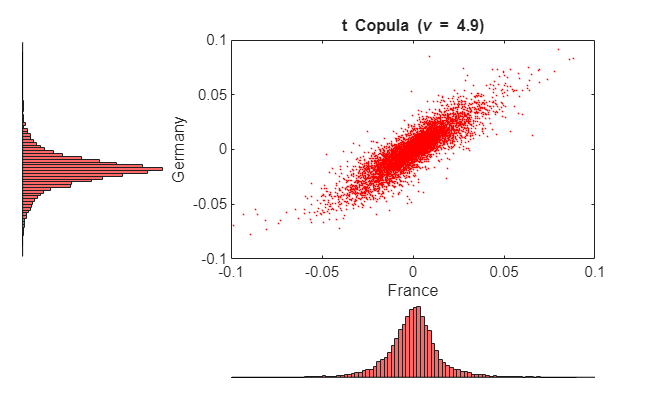Now simulate and plot centered returns using the Gaussian copula.

reset(s) R = zeros(nPoints, nIndices); % pre-allocate simulated returns array U = copularnd('Gaussian', rhoG, nPoints); % simulate U(0,1) from Gaussian copula for j = 1:nIndices R(:,j) = icdf(tails{j}, U(:,j)); end h = scatterhist(R(:,2), R(:,3),'Color','r','Marker','.','MarkerSize',1); fig = gcf; fig.Color = [1 1 1]; y1 = ylim(h(1)); y3 = ylim(h(3)); xlim(h(1), [-.1 .1]) ylim(h(1), [-.1 .1]) xlim(h(2), [-.1 .1]) ylim(h(3), [(y3(1) + (-0.1 - y1(1))) (y3(2) + (0.1 - y1(2)))]) xlabel('France') ylabel('Germany') title('Gaussian Copula')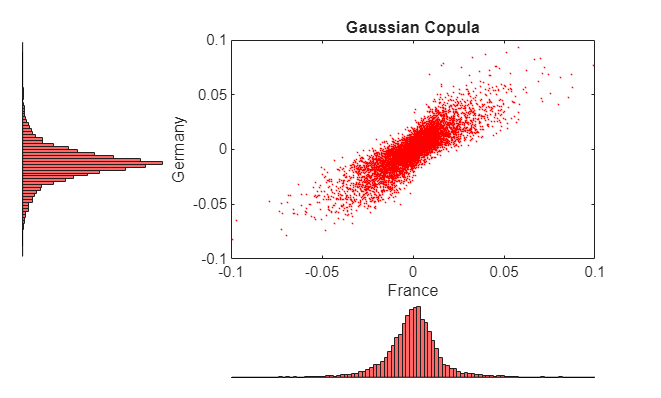Examine these two figures. There is a strong similarity between the miniature histograms on the corresponding axes of each figure. This similarity is not coincidental.

Both copulas simulate uniform random variables, which are then transformed to daily centered returns by the inverse CDF of the piecewise distribution of each index. Therefore, the simulated returns of any given index are identically distributed regardless of the copula.

However, the scatter graph of each figure indicates the dependence structure associated with the given copula, and in contrast to the univariate margins shown in the histograms, the scatter graphs are distinct.

Once again, the copula defines a dependence structure regardless of its margins, and therefore offers many features not limited to calibration alone.

For reference, simulate and plot centered returns using the Gaussian distribution, which underlies the traditional Brownian motion model.

reset(s) R = mvnrnd(zeros(1,nIndices), cov(returns), nPoints); h = scatterhist(R(:,2), R(:,3),'Color','r','Marker','.','MarkerSize',1); fig = gcf; fig.Color = [1 1 1]; y1 = ylim(h(1)); y3 = ylim(h(3)); xlim(h(1), [-.1 .1]) ylim(h(1), [-.1 .1]) xlim(h(2), [-.1 .1]) ylim(h(3), [(y3(1) + (-0.1 - y1(1))) (y3(2) + (0.1 - y1(2)))]) xlabel('France') ylabel('Germany') title('Gaussian Distribution')### American Option Pricing Using the Longstaff & Schwartz Approach

Now that the copulas have been calibrated, compare the prices of at-the-money American basket options derived from various approaches. To simply the analysis, assume that:

• All indices begin at 100.

• The portfolio holds a single unit, or share, of each index such that the value of the portfolio at any time is the sum of the values of the individual indices.

• The option expires in 3 months.

• The information derived from the daily data is annualized.

• Each calendar year is composed of 252 trading days.

• Index levels are simulated daily.

• The option may be exercised at the end of every trading day and approximates the American option as a Bermudan option.

Now compute the parameters common to all simulation methods:

dt = 1 / 252; % time increment = 1 day = 1/252 years yields = Data(:,end); % daily effective yields yields = 360 * log(1 + yields); % continuously-compounded, annualized yields r = mean(yields); % historical 3M Euribor average X = repmat(100, nIndices, 1); % initial state vector strike = sum(X); % initialize an at-the-money basket nTrials = 100; % # of independent trials nPeriods = 63; % # of simulation periods: 63/252 = 0.25 years = 3 months 

Now create two separable multi-dimensional market models in which the riskless return and volatility exposure matrices are both diagonal.

While both are diagonal GBM models with identical risk-neutral returns, the first is driven by a correlated Brownian motion and explicitly specifies the sample linear correlation matrix of centered returns. This correlated Brownian motion process is then weighted by a diagonal matrix of annualized index volatilities or standard deviations.

As an alternative, the same model could be driven by an uncorrelated Brownian motion (standard Brownian motion) by specifying correlation as an identity matrix, or by simply accepting the default value. In this case, the exposure matrix sigma is specified as the lower Cholesky factor of the index return covariance matrix. Because the copula-based approaches simulate dependent random numbers, the diagonal exposure form is chosen for consistency. For further details, see Alternatives for Inducing Dependence & Correlation.

sigma = std(returns) * sqrt(252); % annualized volatility correlation = corrcoef(returns); % correlated Gaussian disturbances GBM1 = gbm(diag(r(ones(1,nIndices))), diag(sigma), 'StartState', X, ... 'Correlation' , correlation); 

Now create the second model driven by the Brownian copula with an identity matrix sigma.

GBM2 = gbm(diag(r(ones(1,nIndices))), eye(nIndices), 'StartState', X); 

The newly created model may seem unusual, but it highlights the flexibility of the SDE architecture.

When working with copulas, it is often convenient to allow the random number generator function Z(t,X) to induce dependence (of which the traditional notion of linear correlation is a special case) with the copula, and to induce magnitude or scale of variation (similar to volatility or standard deviation) with the semi-parametric CDF and inverse CDF transforms. Since the CDF and inverse CDF transforms of each index inherit the characteristics of historical returns, this also explains why the returns are now centered.

In the following sections, statements like:

z = Example_CopulaRNG(returns * sqrt(252), nPeriods, 'Gaussian');

or

z = Example_CopulaRNG(returns * sqrt(252), nPeriods, 't');

fit the Gaussian and t copula dependence structures, respectively, and the semi-parametric margins to the centered returns scaled by the square root of the number of trading days per year (252). This scaling does not annualize the daily centered returns. Instead, it scales them such that the volatility remains consistent with the diagonal annualized exposure matrix sigma of the traditional Brownian motion model (GBM1) created previously.

In this example, you also specify an end-of-period processing function that accepts time followed by state (t,X), and records the sample times and value of the portfolio as the single-unit weighted average of all indices. This function also shares this information with other functions designed to price American options with a constant riskless rate using the least squares regression approach of Longstaff & Schwartz.

f = Example_LongstaffSchwartz(nPeriods, nTrials) 
f = struct with fields: LongstaffSchwartz: @Example_LongstaffSchwartz/saveBasketPrices CallPrice: @Example_LongstaffSchwartz/getCallPrice PutPrice: @Example_LongstaffSchwartz/getPutPrice Prices: @Example_LongstaffSchwartz/getBasketPrices 

Now simulate independent trials of equity index prices over 3 calendar months using the default simByEuler method. No outputs are requested from the simulation methods; in fact, the simulated prices of the individual indices which comprise the basket are unnecessary. Call option prices are reported for convenience:

reset(s) simByEuler(GBM1, nPeriods, 'nTrials' , nTrials, 'DeltaTime', dt, ... 'Processes', f.LongstaffSchwartz); BrownianMotionCallPrice = f.CallPrice(strike, r); BrownianMotionPutPrice = f.PutPrice (strike, r); reset(s) z = Example_CopulaRNG(returns * sqrt(252), nPeriods, 'Gaussian'); f = Example_LongstaffSchwartz(nPeriods, nTrials); simByEuler(GBM2, nPeriods, 'nTrials' , nTrials, 'DeltaTime', dt, ... 'Processes', f.LongstaffSchwartz, 'Z', z); GaussianCopulaCallPrice = f.CallPrice(strike, r); GaussianCopulaPutPrice = f.PutPrice (strike, r); 

Now repeat the copula simulation with the t copula dependence structure. You use the same model object for both copulas; only the random number generator and option pricing functions need to be re-initialized.

reset(s) z = Example_CopulaRNG(returns * sqrt(252), nPeriods, 't'); f = Example_LongstaffSchwartz(nPeriods, nTrials); simByEuler(GBM2, nPeriods, 'nTrials' , nTrials, 'DeltaTime', dt, ... 'Processes', f.LongstaffSchwartz, 'Z', z); tCopulaCallPrice = f.CallPrice(strike, r); tCopulaPutPrice = f.PutPrice (strike, r); 

Finally, compare the American put and call option prices obtained from all models.

disp(' ') fprintf(' # of Monte Carlo Trials: %8d\n' , nTrials) fprintf(' # of Time Periods/Trial: %8d\n\n' , nPeriods) fprintf(' Brownian Motion American Call Basket Price: %8.4f\n' , BrownianMotionCallPrice) fprintf(' Brownian Motion American Put Basket Price: %8.4f\n\n', BrownianMotionPutPrice) fprintf(' Gaussian Copula American Call Basket Price: %8.4f\n' , GaussianCopulaCallPrice) fprintf(' Gaussian Copula American Put Basket Price: %8.4f\n\n', GaussianCopulaPutPrice) fprintf(' t Copula American Call Basket Price: %8.4f\n' , tCopulaCallPrice) fprintf(' t Copula American Put Basket Price: %8.4f\n' , tCopulaPutPrice) 
 # of Monte Carlo Trials: 100 # of Time Periods/Trial: 63 Brownian Motion American Call Basket Price: 25.9456 Brownian Motion American Put Basket Price: 16.4132 Gaussian Copula American Call Basket Price: 24.5711 Gaussian Copula American Put Basket Price: 17.4229 t Copula American Call Basket Price: 22.6220 t Copula American Put Basket Price: 20.9983 

This analysis represents only a small-scale simulation. If the simulation is repeated with 100,000 trials, the following results are obtained:

 # of Monte Carlo Trials: 100000 # of Time Periods/Trial: 63
Brownian Motion American Call Basket Price: 20.2214 Brownian Motion American Put Basket Price: 16.5355
Gaussian Copula American Call Basket Price: 20.6097 Gaussian Copula American Put Basket Price: 16.5539
 t Copula American Call Basket Price: 21.1273 t Copula American Put Basket Price: 16.6873

Interestingly, the results agree closely. Put option prices obtained from copulas exceed those of Brownian motion by less than 1%.

### A Note on Volatility and Interest Rate Scaling

The same option prices could also be obtained by working with unannualized (in this case, daily) centered returns and riskless rates, where the time increment dt = 1 day rather than 1/252 years. In other words, portfolio prices would still be simulated every trading day; the data is simply scaled differently.

Although not executed, and by first resetting the random stream to its initial internal state, the following code segments work with daily centered returns and riskless rates and produce the same option prices.

Gaussian Distribution/Brownian Motion & Daily Data:

reset(s) 
f = Example_LongstaffSchwartz(nPeriods, nTrials); GBM1 = gbm(diag(r(ones(1,nIndices))/252), diag(std(returns)), 'StartState', X, ... 'Correlation', correlation); 
simByEuler(GBM1, nPeriods, 'nTrials' , nTrials, 'DeltaTime', 1, ... 'Processes', f.LongstaffSchwartz); 
BrownianMotionCallPrice = f.CallPrice(strike, r/252) BrownianMotionPutPrice = f.PutPrice (strike, r/252) 

Gaussian Copula & Daily Data:

reset(s) 
z = Example_CopulaRNG(returns, nPeriods, 'Gaussian'); f = Example_LongstaffSchwartz(nPeriods, nTrials); GBM2 = gbm(diag(r(ones(1,nIndices))/252), eye(nIndices), 'StartState', X); 
simByEuler(GBM2, nPeriods, 'nTrials' , nTrials, 'DeltaTime', 1, ... 'Processes', f.LongstaffSchwartz , 'Z', z); 
GaussianCopulaCallPrice = f.CallPrice(strike, r/252) GaussianCopulaPutPrice = f.PutPrice (strike, r/252) 

t Copula & Daily Data:

reset(s) 
z = Example_CopulaRNG(returns, nPeriods, 't'); f = Example_LongstaffSchwartz(nPeriods, nTrials); 
simByEuler(GBM2, nPeriods, 'nTrials' , nTrials, 'DeltaTime', 1, ... 'Processes', f.LongstaffSchwartz , 'Z', z); 
tCopulaCallPrice = f.CallPrice(strike, r/252) tCopulaPutPrice = f.PutPrice (strike, r/252) 

Download ebook